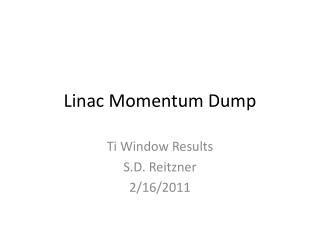Download PresentationLinac Momentum Dump

# Linac Momentum Dump

Download Presentation## Linac Momentum Dump

- - - - - - - - - - - - - - - - - - - - - - - - - - - E N D - - - - - - - - - - - - - - - - - - - - - - - - - - -
##### Presentation Transcript

1. Linac Momentum Dump Ti Window Results S.D. Reitzner 2/16/2011

2. Linac Input Params • 400 MeV protons • Vertex • 350 cm upstream from wall • Stainless steel pipe 6, 8” diameter • Ti 6Al-4V window 10, 15 mils • 5’, 4’, 2’, -10” from wall

3. Beam Window Side View 5’ Sleeve

4. No Window Total Flux (cm-2 s-1)

5. R = 6” Z = 5’ dZ = 10 mils Fluence

6. R = 8” Z = 5’ dZ = 10 mils Fluence

7. R = 8” Z = 5’ dZ = 15 mils Fluence

8. R = 6” Z = 4’ dZ = 10 mils Fluence

9. R = 6” Z = 2’ dZ = 10 mils Fluence

10. Z = -10” dZ = 10 mils Fluence

11. Z = -10” dZ = 15 mils Fluence

12. No Window Dose Equivalent (mSv/hr)

13. R = 6” Z = 5’ dZ = 10 mils Dose Equivalent (mSv/h)

14. R = 8” Z = 5’ dZ = 10 mils Dose Equivalent (mSv/h)

15. R = 8” Z = 5’ dZ = 15 mils Dose Equivalent (mSv/h)

16. R = 6” Z = 4’ dZ = 10 mils Dose Equivalent (mSv/h)

17. R = 6” Z = 2’ dZ = 10 mils Dose Equivalent (mSv/h)

18. Z = -10” dZ = 10 mils Dose Equivalent (mSv/h)

19. Z = -10” dZ = 15 mils Dose Equivalent (mSv/hr)

20. No Window Dose Residue (mSv/hr)

21. R = 6” Z = 5’ dZ = 10 mils Dose Residual (mSv/hr)

22. R = 8” Z = 5’ dZ = 10 mils Dose Residual (mSv/hr)

23. R = 8” Z = 5’ dZ = 15 mils Dose Residual (mSv/hr)

24. R = 6” Z = 4’ dZ = 10 mils Dose Residual

25. R = 6” Z = 2’ dZ = 10 mils Dose Residual

26. Z = -10” dZ = 10 mils Dose Residual (mSv/hr)

27. Z = -10” dZ = 15 mils Dose Residual (mSv/hr)

28. Star Density No Window

29. R = 6” Z = 5’ dZ = 10 mils Star Density

30. R = 8” Z = 5’ dZ = 10 mils Star Density

31. R = 8” Z = 5’ dZ = 15 mils Star Density

32. R = 6” Z = 4’ dZ = 10 mils Star Density

33. R = 6” Z = 2’ dZ = 10 mils Star Density

34. Z = -10” dZ = 10 mils Star Density

35. Z = -10” dZ = 15 mils Star Density